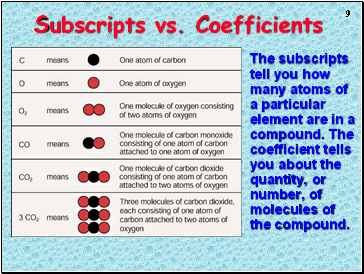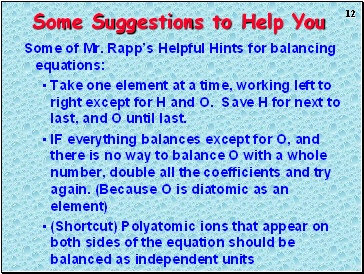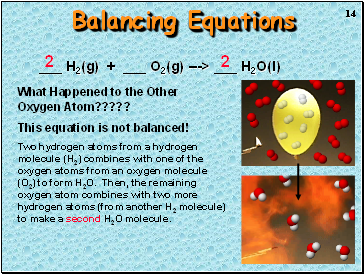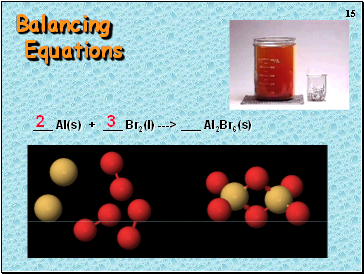# Chemical reactions IPage 2

#### WATCH ALL SLIDES

Changing the subscripts changes the compound. Subscripts are determined by the valence electrons (charges for ionic or sharing for covalent)

Slide 9Subscripts vs. Coefficients

The subscripts tell you how many atoms of a particular element are in a compound. The coefficient tells you about the quantity, or number, of molecules of the compound.

Slide 10Chemical Equations

4 Al(s) + 3 O2(g) ---> 2 Al2O3(s)

This equation means

4 Al atoms + 3 O2 molecules ---produces--->

2 molecules of Al2O3 AND/OR

4 moles of Al + 3 moles of O2 ---produces--->

2 moles of Al2O3

Slide 11## Steps to Balancing Equations

There are four basic steps to balancing a chemical equation.

Write the correct formula for the reactants and the products. DO NOT TRY TO BALANCE IT YET! You must write the correct formulas first. And most importantly, once you write them correctly DO NOT CHANGE THE FORMULAS!

Find the number of atoms for each element on the left side. Compare those against the number of the atoms of the same element on the right side.

Determine where to place coefficients in front of formulas so that the left side has the same number of atoms as the right side for EACH element in order to balance the equation.

The numbers of atoms on both sides of the equation are now balanced.

The coefficients are in the lowest possible whole number ratios. (reduced)

Slide 12Some of Mr. Rapp’s Helpful Hints for balancing equations:

Take one element at a time, working left to right except for H and O. Save H for next to last, and O until last.

IF everything balances except for O, and there is no way to balance O with a whole number, double all the coefficients and try again. (Because O is diatomic as an element)

(Shortcut) Polyatomic ions that appear on both sides of the equation should be balanced as independent units

Slide 13Slide 14Balancing Equations

_ H2(g) + _ O2(g) ---> _ H2O(l)

2

2

What Happened to the Other Oxygen Atom?????

This equation is not balanced!

Two hydrogen atoms from a hydrogen molecule (H2) combines with one of the oxygen atoms from an oxygen molecule (O2) to form H2O. Then, the remaining oxygen atom combines with two more hydrogen atoms (from another H2 molecule) to make a second H2O molecule.

Slide 15Go to page:
1  2  3